# Ac Through Inductor Only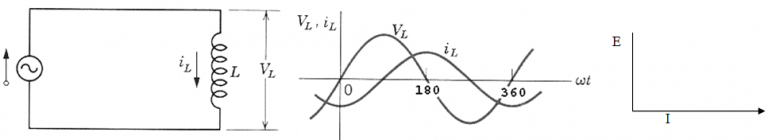Let an alternating emf be applied to a circuit containing inductor only such type of circuit is called inductive circuit.
Let the emf applied to the circuit is: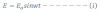Then the induced emf across the inductor is: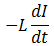This emf opposes the growth of current in the circuit. Applying kirchhoff’s voltage law in the loop of fig a,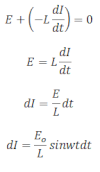Integrating above expression we get,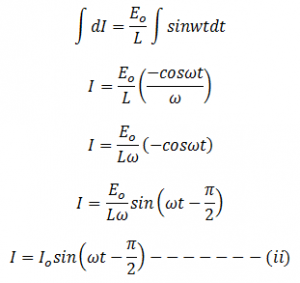This is the form of alternating current developed in the purely inductive circuit. Equation i and ii shows that in a purely inductive circuit alternating emf leads the alternating current by π/2.